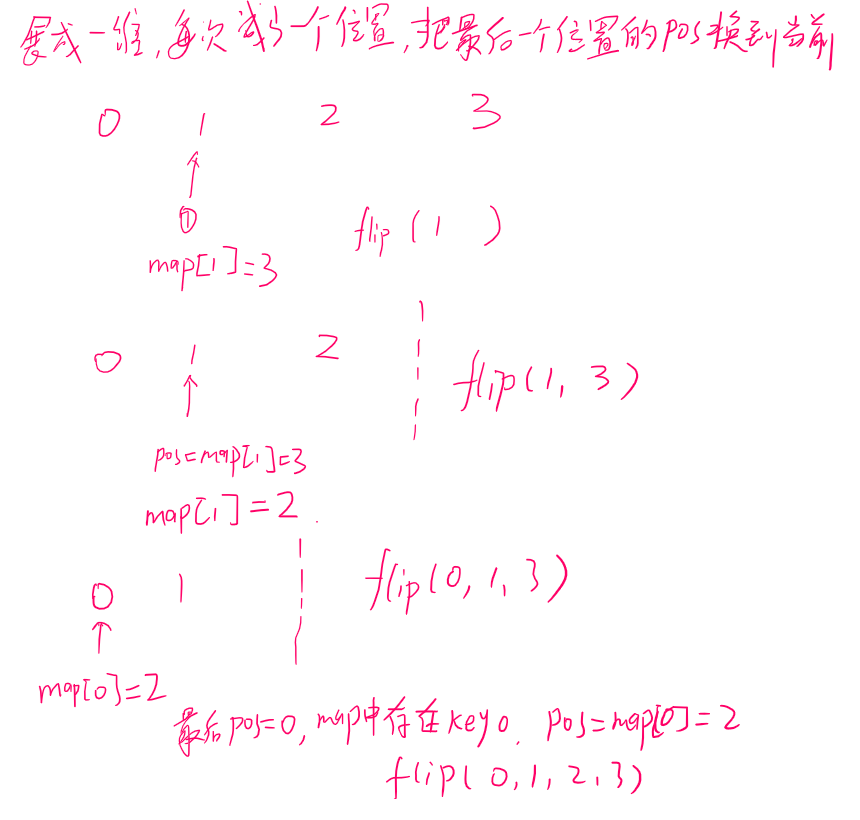# LeetCode 519. 随机翻转矩阵（哈希）

## 1. 题目

注意:
1 <= n_rows, n_cols <= 10000
0 <= row.id < n_rows 并且 0 <= col.id < n_cols

["Solution","flip","flip","flip","flip"]
[[2,3],[],[],[],[]]

["Solution","flip","flip","reset","flip"]
[[1,2],[],[],[],[]]

Solution 的构造函数有两个参数，分别为 n_rows 和 n_cols。
flip 和 reset 没有参数，参数总会以列表形式给出，哪怕该列表为空


## 2. 解题

### 2.1 超时解

• 矩阵很大的时候，翻得时候效率很低，会碰到翻过的，还要去重新翻
class Solution {    //超时
vector<int> grid;
int m, n;
int x, y, pos;
public:
Solution(int n_rows, int n_cols) {
grid = vector<int> (n_rows*n_cols, 0);
m = n_rows;
n = n_cols;
}

vector<int> flip() {
do
{
pos = rand()%(m*n);
}while(grid[pos] == 1);
grid[pos] = 1;
return {pos/n, pos-pos/n*n};
}

void reset() {
grid = vector<int> (m*n, 0);
}
};


### 2.2 转一维，每次缩小范围

• 记录总共的元素个数N，随机获取 0 ~ N-1 的 pos
• 如果map中有key = pos，则 pos = map[pos]，如果没有，pos就是pos
• 还需要把当前取的位置的 map的 value 更新为最后一个位置的，下一轮，最后那个位置就跳过了class Solution {
unordered_map<int,int> map;
int m, n, num;
int x, y, pos, prev;
public:
Solution(int n_rows, int n_cols) {
m = n_rows;
n = n_cols;
num = m*n;
}

vector<int> flip() {
if(num == 0) return {};
pos = rand()%(num);
num--;//下一轮，减少一个数
if(map.count(pos))//map包含pos的key
{
prev = pos;//记录当前pos
pos = map[pos];//真实的取走的pos
if(!map.count(num))//把最后一个位置的数换到当前
map[prev] = num;
else//如果最后一个位置有map值，用其值替换
map[prev] = map[num];
}
else//map不包含pos的key
{	//pos就是当前位置，只需把末尾的数替换到当前，同上
if(!map.count(num))
map[pos] = num;
else
map[pos] = map[num];
}
x = pos/n;
y = pos-x*n;
return {x, y};
}

void reset() {
num = m*n;
map.clear();
}
};


36 ms 18.6 MB

03-0749

#### 【leetcode】519. Random Flip Matrix

04-07306

#### LeetCode 随机翻转矩阵12-26131

#### 519. 随机翻转矩阵

10-1254

#### [LeetCode] Random Flip Matrix 随机翻转矩阵

12-18423

#### Leetcode:519. 随机翻转矩阵

07-1514

#### Leetcode 519. 随机翻转矩阵 C++

04-26465

#### www.leetcode.com

09-065万+

#### LeetCode解题汇总目录

06-26782

#### LeetCode 1072. 按列翻转得到最大值等行数（查找相同的模式，哈希计数）

11-1876

#### 随机数索引©️2020 CSDN 皮肤主题: 代码科技 设计师: Amelia_0503点击重新获取扫码支付1.余额是钱包充值的虚拟货币，按照1:1的比例进行支付金额的抵扣。
2.余额无法直接购买下载，可以购买VIP、C币套餐、付费专栏及课程。余额充值